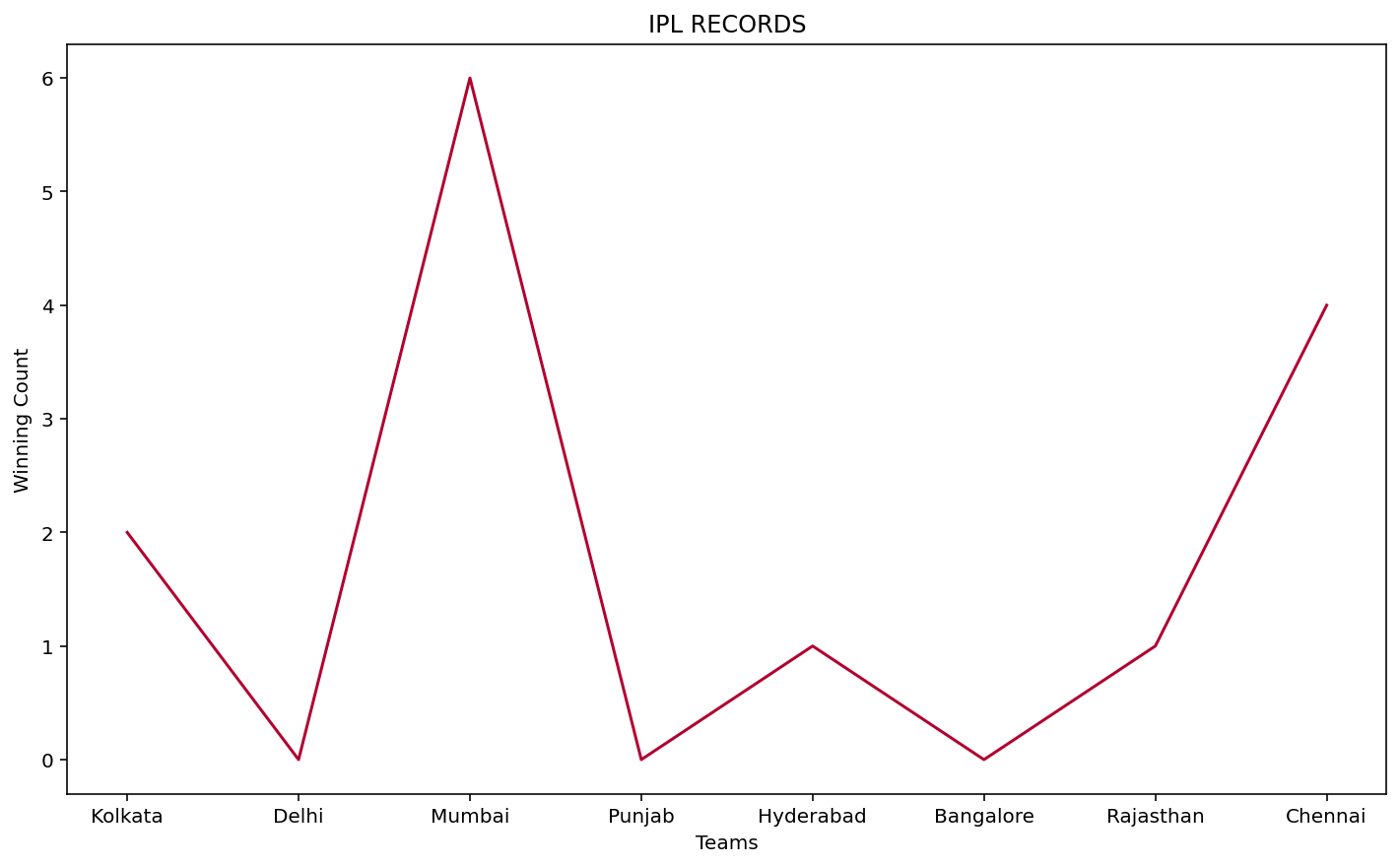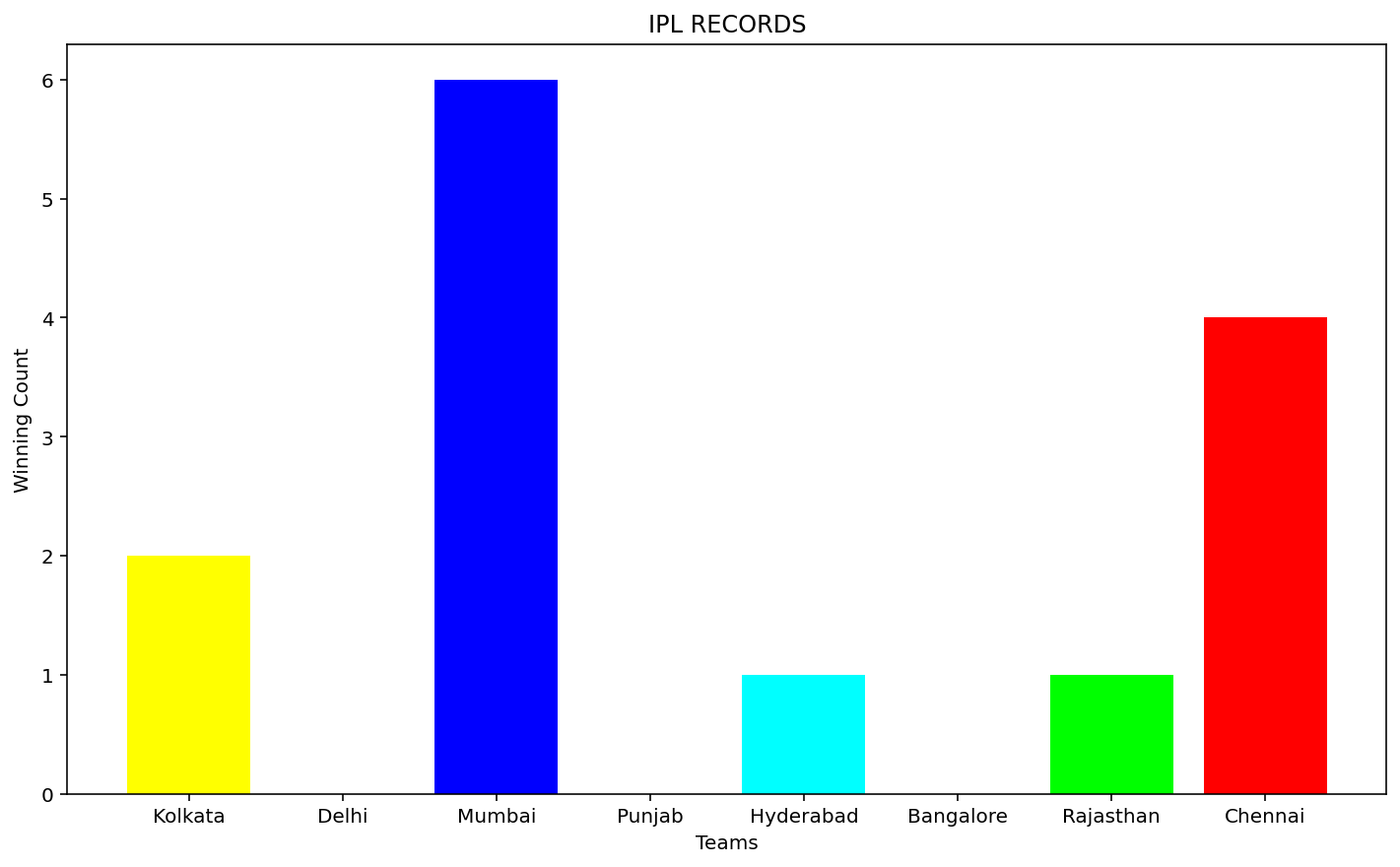# How to generate a random color for a Matplotlib plot in Python?

• Last Updated : 11 Dec, 2020

Handling huge dataset requires libraries and methods that can be used to store, analyze and manipulate the datasets. Python is a popular choice when it comes to data science and analytics. Data scientists prefer Python due to its wide library support that contains functions which can be used to work with datasets to create graphs and charts. Matplotlib is a data visualization library in Python. Matplotlib works similar to Matlab and produces interactive charts and graphs. Matplotlib offers variety of chart types to choose from. The chart properties can be set explicitly using the inbuilt methods and attributes. To generate random colors for a Matplotlib plot in Python the matplotlib.pyplot and random libraries of Python are used. Following is an example to generate random colors for a Matplotlib plot :

First Approach

1. A dataset is created with a teams array and wincount array.
2. The teams array is plotted against the X axis and wincount array is plotted against the Y axis. Matplotlib supports RGB or RGBA with float values in the range 0-1.
3. Now RGB or RGBA values that range between 0-1 determine the color for the chart.
4. In this example, in-order to create random values ranging between 0 and 1. The red ‘r’, green ‘g’ and blue ‘b’ values obtained are then passed into a tuple color which forms the final color.
5. This tuple is next assigned to the color attribute of plot() method.
6. The X and Y axis are labeled and a title is provided to the chart using the xlabel() , ylabel() and title() method of matplotlib.pyplot library.

Code Implementation:

## Python3

 `import` `random as random``import` `matplotlib.pyplot as plt`` ` `teams ``=` `[``'Kolkata'``, ``'Delhi'``, ``'Mumbai'``, ``'Punjab'``,``         ``'Hyderabad'``, ``'Bangalore'``, ``'Rajasthan'``, ``'Chennai'``]`` ` `wincount ``=` `[``2``, ``0``, ``6``, ``0``, ``1``, ``0``, ``1``, ``4``]`` ` `r ``=` `random.random()``b ``=` `random.random()``g ``=` `random.random()`` ` `color ``=` `(r, g, b)`` ` `plt.xlabel(``"Teams"``)``plt.ylabel(``"Winning Count"``)``plt.title(``"IPL RECORDS"``)``plt.plot(teams, wincount, c``=``color)`

Output:Second Approach

1. In the second approach, a bar chart is demonstrated. An empty list is declared to hold the color tuples.
2. The X and Y axis are labeled and a title is set for the chart.
3. To append different colors for n records a for loop is executed.
4. The random.choice() method of numpy library is used to create tuples of size 3 with values between 0 and 1.
5. The teams and wincount array are plotted against the X and Y axis.
6. The color attribute of bar() method of matplotlib.pyplot is assigned the list of tuples. Random tuples are chosen from the list for each bar.

Code Implementation

## Python3

 `import` `random as random``import` `matplotlib.pyplot as plt``import` `numpy as np`` ` `l ``=` `[]``teams ``=` `[``'Kolkata'``, ``'Delhi'``, ``'Mumbai'``, ``'Punjab'``,``         ``'Hyderabad'``, ``'Bangalore'``, ``'Rajasthan'``, ``'Chennai'``]`` ` `wincount ``=` `[``2``, ``0``, ``6``, ``0``, ``1``, ``0``, ``1``, ``4``]`` ` `plt.xlabel(``"Teams"``)``plt.ylabel(``"Winning Count"``)``plt.title(``"IPL RECORDS"``)`` ` `for` `i ``in` `range``(``0``, ``len``(teams)``+``1``):``    ``l.append(``tuple``(np.random.choice(``range``(``0``, ``2``), size``=``3``)))``     ` `plt.bar(teams, wincount, color``=``l)`

Output:However the disadvantage of using random colors is in case white is chosen, the particular bar or line becomes invisible.

My Personal Notes arrow_drop_up